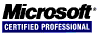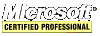# JavaScript Tutorial

### The Array Type

Arrays can be created in two ways. The first is to use the Array constructor:

``var animals = new Array();``
``var animals = new Array(3); // array with 3 elements``
``var animals = new Array("cat", "dog", "bird");``

The second way to create an array is by using array literal notation.

``````var animals = ["cat", "dog", "bird"];
var values = []; // creates an empty array``````

Use the `length` property to discover the size of the array.

``````var animals = ["cat", "dog", "bird"];
var values = [];

The length property is helpful in adding items to the end of an array.

``````var values = [];
values[values.length] = 12;
values[values.length] = 15;
values[values.length] = 18;``````

#### Detecting Arrays

Use the `Array.isArray()` method to discover if it is an array.

``````if (!Array.isArray) {
Array.isArray = function(arg) {
return Object.prototype.toString.call(arg) == "[object Array]";
};
}
if (Array.isArray(value)) {
// some array related code
}``````

#### Conversion Methods

All objects (which includes arrays) have `toLocalString()`, `toString()` and `valueOf()` methods. Concerning arrays, the `toString()` and `valueOf()` methods return the same value, which is a comma-separated string that contains the string equivalents of each value in the array.

``````var animals = ["cat", "dog", "bird"];

#### Stack Methods

An array object can act just like a stack, which is one of a group of data structures that restrict the insertion and removal of items. The most recently added item is the first one removed. The insertion (called a push) and removal (called a pop) of items in a stack occur at the top of the stack. Arrays provide `push()` and `pop()` specifically to allow stack-like behavior.

``````var animals = new Array();				// create an array
var count = animals.push("cat", "dog");	 // push two items on the stack

count = animals.push("bird");			 // push another item on the stack

var item = animals.pop();				 // get the last item from the stack

#### Queue Methods

A queue adds items to the end of a list and retrieves items from the front of the list. All that is needed to emulate a queue is a method to retrieve the first item in the array because the `push()` method adds items to the end of an array. The array method for this is called `shift()`, which removes the first item in the array and returns it, decrementing the length of the array by one.

``````var animals = new Array();				// create an array
var count = animals.push("cat", "dog");	 // push two items into the queue

count = animals.push("bird");			 // push another item into the queue

var item = animals.shift();				 // get the first item in the queue

JavaScript also provides an `unshift()` method for arrays. It is possible to emulate a queue in the opposite direction, so that new values are added to the front of the array and values are retrieved off the back, by using `unshift()` in combination with `pop()`.

``````var colors = new Array();				 // create an array
var count = colors.unshift("cat", "dog"); // push two items into the queue

count = colors.unshift("bird");			 // push another item into the queue

var item = colors.pop();					// get the first item in the queue

#### Reordering Methods

The `reverse()` and `sort()` methods deal directly with the reordering of items already in an array.

``````var values = [5, 4, 3, 2, 1];
values.reverse();

By default, the `sort()` method puts the items in ascending order by converting the values to strings.

``````var values = [0, 1, 6, 11, 13];
values.sort();
alert(values);					// shows "0,1,11,13,6" — not what one would expect``````

Obviously, this is not optimal in many cases, so the `sort()` method allows one to pass in a comparison function indicating which value should come before which.

``````function compareAscending(value1, value2) {
if (value1 < value2) {
return -1;
}
else if (value1 > value2) {
return 1;
}
return 0;
}
function compareDescending(value1, value2) {
if (value1 < value2) {
return 1;
}
else if (value1 > value2) {
return -1;
}
return 0;
}
var values = [0, 13, 11, 1, 6];
values.sort(compareAscending);
A simpler version of the comparison function can be used with numeric types and objects whose `valueOf()` method returns numeric values such as the `Date` object.
``````function compare(value1, value2){